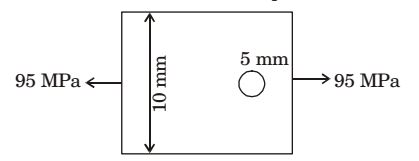## Machine Design Miscellaneous

#### Machine Design

1. A ductile material having an endurance limit of 196 N/mm2 and the yield point at 294 N/ mm2 is stressed under variable load. The maximum and minimum stresses are 147 N/ mm2 and 49 N/mm2. The fatigue stress concentration factor is 1.32. The available factor of safety for this loading is

1. Consider Soderberg equation

 τa + τm = 1 Se Sy FOS

 τa = 147 - 49 = 49 MPa 2

 τm = 147 + 49 = 98 MPa 2

 Se = 196 = 147.48 MPa 1.32

 49 + 98 = 1 147.48 294 FOS

FOS = 1.5

##### Correct Option: B

Consider Soderberg equation

 τa + τm = 1 Se Sy FOS

 τa = 147 - 49 = 49 MPa 2

 τm = 147 + 49 = 98 MPa 2

 Se = 196 = 147.48 MPa 1.32

 49 + 98 = 1 147.48 294 FOS

FOS = 1.5

1. A solid shaft can resist a bending moment of 3.0 kNm and a twisting moment of 4.0 kNm together, then the maximum torque that can be applied is

1. Maximum tensile stress due to bending,

 ft = M Z

 = 3 × 103 {(π / 64 ) × d4 } d / 2

 ft = 30.558 kPa d3

 Maximum shear stress due to torsion, fs = TR J

 = 4 × 103 × (d / 2) π × d4 32

 fs = 20.7 kPa d3

When both the stress acts simultaneously, maximum induced shear stress,
 fs max. = 1 [√(ft)² + 4(fs)² ] 2

Maximum torque that can be applied,
 T = πd3 fs max. 16

 = πd3 × 1 [ √(30.558 / d3)² + 4(20.37 / d3)² ] 16 2

= 4 kNm

##### Correct Option: D

Maximum tensile stress due to bending,

 ft = M Z

 = 3 × 103 {(π / 64 ) × d4 } d / 2

 ft = 30.558 kPa d3

 Maximum shear stress due to torsion, fs = TR J

 = 4 × 103 × (d / 2) π × d4 32

 fs = 20.7 kPa d3

When both the stress acts simultaneously, maximum induced shear stress,
 fs max. = 1 [√(ft)² + 4(fs)² ] 2

Maximum torque that can be applied,
 T = πd3 fs max. 16

 = πd3 × 1 [ √(30.558 / d3)² + 4(20.37 / d3)² ] 16 2

= 4 kNm

1. Two shafts A and B are made of the same material. The diameter of shaft B is twice that of shaft A The ratio of power which can be transmitted by shaft A to that of shaft B is

1.  TA = π × d3τ 16

 TB = π × (2d)3τ 16

 PA = TA = 1 PB TB 8

##### Correct Option: C

 TA = π × d3τ 16

 TB = π × (2d)3τ 16

 PA = TA = 1 PB TB 8

1. The outside diameter of a hollow shaft that is twice its inside diameter the ratio of its torque carrying capacity to that of a solid shaft of the same material and the same outside diameter is

1.  Thollow = πd4 - d42τ ......(1) 32 16 d

 Tsolid = π d3τ .......(2) 16

By dividing (1) with (2)
 Thollow = 15 Tsolid 16

##### Correct Option: A

 Thollow = πd4 - d42τ ......(1) 32 16 d

 Tsolid = π d3τ .......(2) 16

By dividing (1) with (2)
 Thollow = 15 Tsolid 16

1. A large uniform plate containing a rivet hole subjected to uniform uniaxial tension of 95 MPa. The maximum stress in the plate is1.  Max Stress = Avg. Force Min. Area

 = 95 × 10 × t = 100 MPa (10 - 0.5)t

##### Correct Option: A

 Max Stress = Avg. Force Min. Area

 = 95 × 10 × t = 100 MPa (10 - 0.5)t